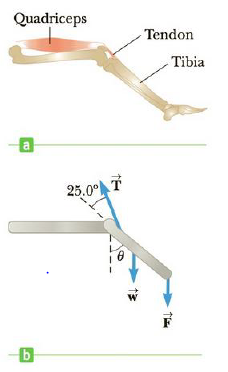Chapter 8, Problem 35P

Chapter
Section
Textbook Problem

The large quadriceps muscle in the upper leg terminates at its lower end in a tendon attached to the upper end of the tibia (Fig. P8.35a). The forces on the lower leg when the leg is extended are modeled as in Figure P8.35b, where T → is the force of tension in the tendon, w → is the force of gravity acting on the lower leg, and F → is the force of gravity acting on the foot. Find T → when the tendon is at an angle of 25.0° with the tibia, assuming that w = 30.0 N,F = 12.5 N, and the leg is extended at an angle θ of 40.0° with the vertical. Assume that, the center of gravity of the lower leg is at its center and that the tendon attaches to the lower leg at a point one-fifth of the way down the leg.Figure P8.35

To determine
The tension in the tendon when it is at an angle of 25.0° with tibia.

Explanation

Given info: The force of gravity acting on the lower leg is 30.0N , the force of gravity acting on the foot is 12.5N , the angle of extended leg with the vertical is 40.0° , and the angle of tension with tibia is 25.0° .

Explanation:

The following figure shows the tension in the tendon.

Assume a coordinate system in which, the x axis of the coordinate is parallel to the tibia and the y axis of the coordinate is perpendicular to it. The sum of torques acting on it is τ=0=(d/2)wydFy+(d/5)Ty and this expression is written as (d/2)wsinθd(Fsinθ)+(d/5)Tsinθ'=0 .

The formula for the tension in the tendon is,

T=5(w2+F)sinθsinθ'

• w is the force of gravity on the lower leg

Still sussing out bartleby?

Check out a sample textbook solution.

See a sample solution

The Solution to Your Study Problems

Bartleby provides explanations to thousands of textbook problems written by our experts, many with advanced degrees!

Get Started